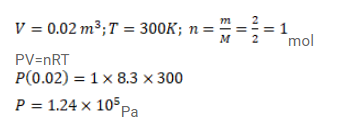# Use R=8.3 J/mol-K wherever required.Question:

Use $\mathrm{R}=8.3 \mathrm{~J} / \mathrm{mol}-\mathrm{K}$ wherever required.

$2 \mathrm{~g}$ of hydrogen is sealed in vessel of volume $0.02 \mathrm{~m}^{3}$ and is aintained at $300 \mathrm{~K}$. Calculate the pressure in the vessel.

Solution: